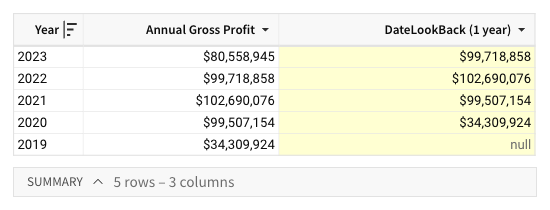# DateLookback

The DateLookback function returns the value of a variable at a previous point in time (or lookback period) determined by a specified date and offset.

## Syntax

`DateLookback(value, date, amount, period)`

Function arguments:

 value (required) The value to look up. Can be a column to reference, a formula to compute, or a constant. When referencing a column, the data must contain unique period values or be aggregated at the same date granularity as the period argument. Non-unique period values can result in null or multiple values output. date (required) The date to reference when offsetting the lookback period. Can be a date, date column, or formula that returns a date. amount (required) The number of periods to offset the lookback period. Can be a positive integer (for lookback) or negative integer (for lookahead). period (required) The unit of time to use for the offset and lookback period. Can be `"year"`, `"quarter"`, `"month"`, `"week"`, `"day"`, `"hour"`, `"minute"`, or `"second"`.

## Example

A table includes an Annual Gross Profit column containing the gross profit for each year between 2019 and 2023. You can use the DateLookback function to return the previous year’s gross profit and facilitate a period-over-period analysis.

`DateLookback([Annual Gross Profit], [Year], 1, "year")`

The formula determines a one-year offset from the period in the Year column, then references the offset period and returns the corresponding value from the Annual Gross Profit column.